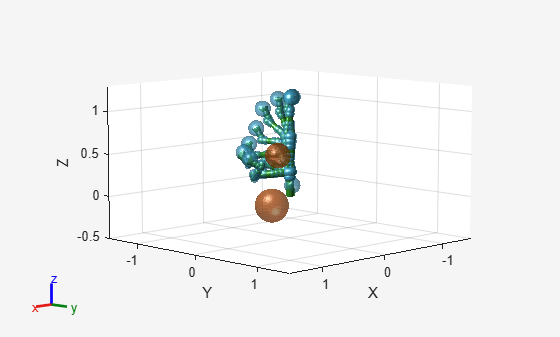# chompSmoothnessOptions

Smoothness options for CHOMP trajectories

Since R2023a

## Description

The `chompSmoothnessOptions` object stores smoothness options that determine how to weight smoothness costs for trajectories generated using Covariant Hamiltonian Optimization for Motion Planning (CHOMP). Use this object to optimize the smoothness of a trajectory generated using CHOMP.

## Creation

### Syntax

``OPTS = chompSmoothnessOptions``
``OPTS = chompSmoothnessOptions(Name=Value)``

### Description

````OPTS = chompSmoothnessOptions` creates a smoothness options object `OPTS` that you can use to optimize the smoothness of a trajectory using CHOMP.```

example

````OPTS = chompSmoothnessOptions(Name=Value)` specifies properties using one or more name-value arguments.```

## Properties

expand all

Weight on the velocity smoothness cost of the trajectory, specified as a nonnegative numeric scalar. This is a weight on the cost obtained by the summation of squared velocity along the trajectory.

Weight on the acceleration smoothness cost of the trajectory, specified as a nonnegative numeric scalar. This is a weight on the cost obtained by the summation of squared acceleration along the trajectory.

Weight on the jerk smoothness cost of the trajectory, specified as a nonnegative numeric scalar. This is a weight on the cost obtained by the summation of squared jerk along the trajectory.

Weight on the overall smoothness of the trajectory, specified as a nonnegative numeric scalar. This is a weight on the cost obtained by the summation of the velocity, jerk, and acceleration smoothness costs.

## Examples

collapse all

Load a robot model into the workspace, and create a CHOMP solver.

```robot = loadrobot("kinovaGen3",DataFormat="row"); chomp = manipulatorCHOMP(robot);```

Create spheres to represent obstacles, and add them to the CHOMP solver.

```env = [0.20 0.2 -0.1 -0.1; % sphere, radius 0.20 at (0.2,-0.1,-0.1) 0.15 0.2 0.0 0.5]'; % sphere, radius 0.15 at (0.2,0.0,0.5) chomp.SphericalObstacles = env;```

To prioritize a collision-free trajectory, set the smoothness cost weight to a lower value than the collision cost weight. Then add the options to the CHOMP solver.

```chomp.SmoothnessOptions = chompSmoothnessOptions(SmoothnessCostWeight=1e-3); chomp.CollisionOptions = chompCollisionOptions(CollisionCostWeight=10); chomp.SolverOptions = chompSolverOptions(Verbosity="none",LearningRate=7.0);```

Initialize a trajectory, optimize it using the CHOMP solver, and show the waypoints in a figure.

```startconfig = homeConfiguration(robot); goalconfig = [0.5 1.75 -2.25 2.0 0.3 -1.65 -0.4]; timepoints = [0 5]; timestep = 0.1; trajtype = "minjerkpolytraj"; [wptsamples,tsamples] = optimize(chomp, ... [startconfig; goalconfig], ... timepoints, ... timestep, ... InitialTrajectoryFitType=trajtype); show(chomp,wptsamples,NumSamples=10); zlim([-0.5 1.3])```Ratliff, Nathan, Siddhartha Srinivasa, Matt Zucker, and Andrew Bagnell. “CHOMP: Gradient Optimization Techniques for Efficient Motion Planning.” In 2009 IEEE International Conference on Robotics and Automation, 489–94. Kobe, Japan: IEEE, 2009. https://doi.org/10.1109/ROBOT.2009.5152817.